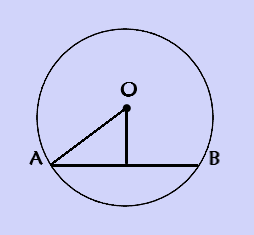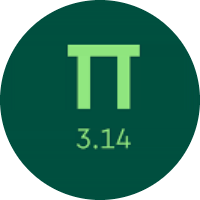# Understanding the Concept of Two Dimensional Figures

In this quiz, you will learn about two-dimensional figures. You will learn about regular and irregular polygons, parallel, perpendicular, intersecting lines, types of angles, and the properties of polygons.

Start Quiz

Is this a polygon?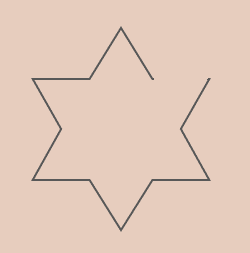Yes

No

Is this a polygon?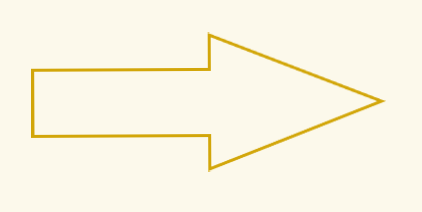Yes

No

Is this shape a regular pentagon?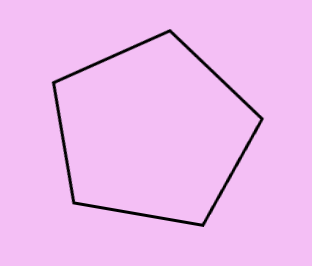Yes

No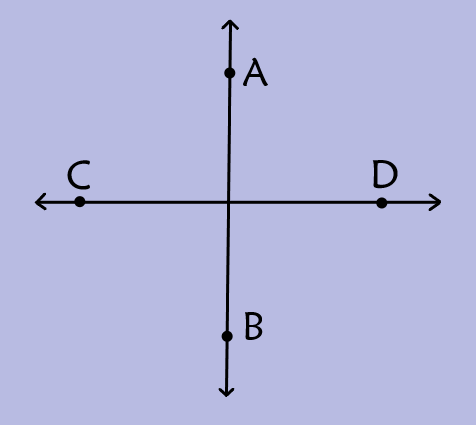In the figure shown, AB and CD are?

Parallel to each other

Perpendicular to each other

Is this angle acute, right, obtuse, or straight?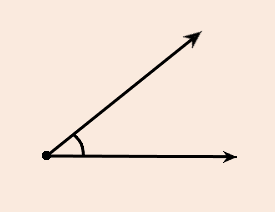Acute

Obtuse

Right

Straight

What fraction of a turn is this angle?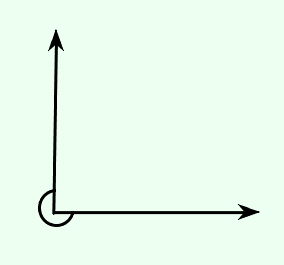1/2

1/4

3/4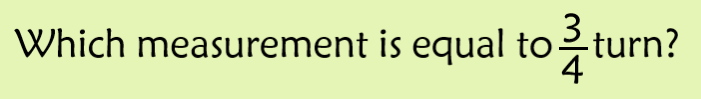90

180

270

360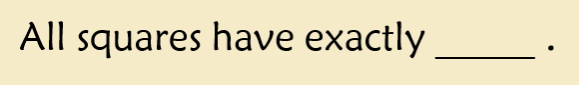A pair of parallel sides

2 acute angles

3 obtuse angles

4 congruent sides

What is OA?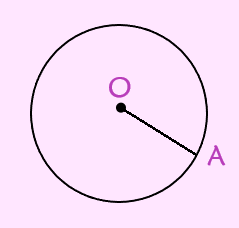Diameter

Chord

What is AB?